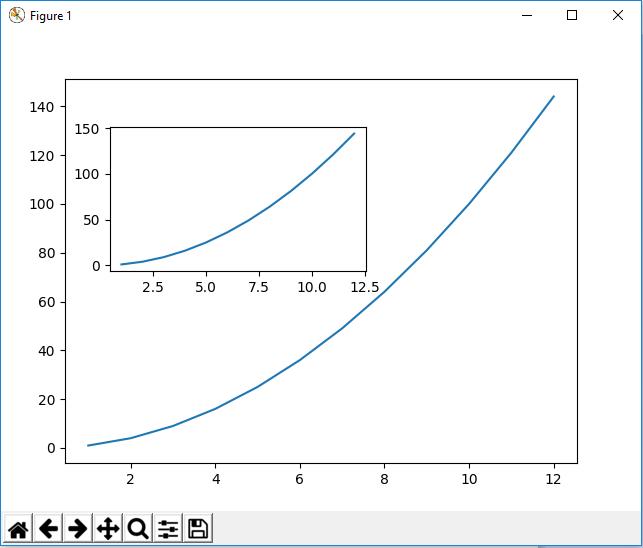﻿ How to Add Multiple Axes to a Figure in Matplotlib with Python ﻿# How to Add Multiple Axes to a Figure in Matplotlib with PythonIn this article, we show how to add multiple axes to a figure in matplotlib with Python.

So with matplotlib, the heart of it is to create a figure.

On this figure, you can populate it with all different types of data, including axes, a graph plot, a geometric shape, etc.

We don't have to simply populate the figure with one X and Y axes.

We can add multiple axes to the figure.

This is shown in the following code below.

So the first thing we have to do is import matplotlib. We do this with the line, import matplotlib.pyplot as plt

We then create a variable fig, and set it equal to, plt.figure()

This creates a figure object, which of course is initially empty, because we haven't populated it with anything.

So we have a figure object stored in the variable, fig, that is empty.

We then add axes to this figure.

Our first axes is the bigger of the axes, axes1.

The line, fig.add_axes([0.1,0.1,0.8,0.8]), makes the figure 10% from the left of the figure, 10% from the bottom of the figure, 80% width of the figure, and 80% height of the figure.

From left to the right, the values of the add_axes() function is [left, bottom, width, height]

We then create a second axes, axes2.

This second axes is created with the line, axes2= fig.add_axes([0.17,0.5,0.4,0.3])

This second axes is created and placed 17% from the left of the figure, 50% from the bottom of the figure, takes up 40% of the width of the figure, and takes up 30% of the height of the figure. This places this axes within the larger axes.

The x axis is composed of the values 1-12.

The y axis is compsed of the values 12-144.

We then plot graphs of the x and y values on each of the axes.

To show this figure object, we use the line, fig.show()

This works if you're using a python IDE other than jupyter notebooks. If you are using jupyter notebooks, then you would not use, plt.show(). Instead you would specify in the code right after importing matplotlib, %matplotlib inline

This line allows the figure of a graph to be shown with jupyter notebooks.

After running the following code above, we get the following figure with axes shown in the image below.So now you see a figure object with axes added to it.

And this is how to add axes to an figure object in matplotlib with Python.

Related Resources

How to Randomly Select From or Shuffle a List in Python